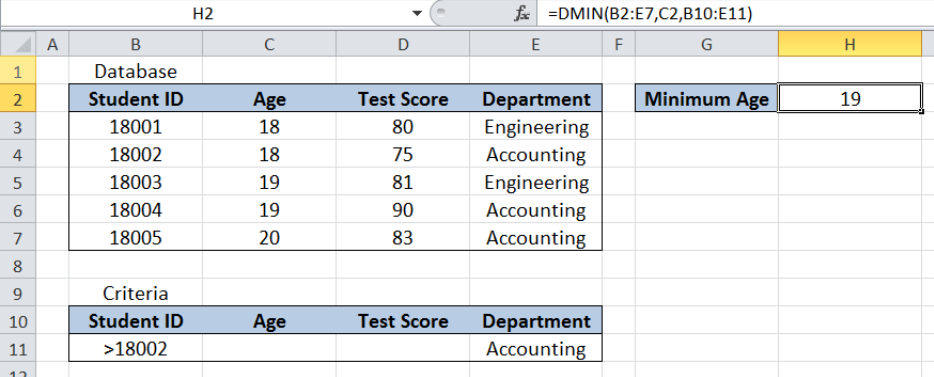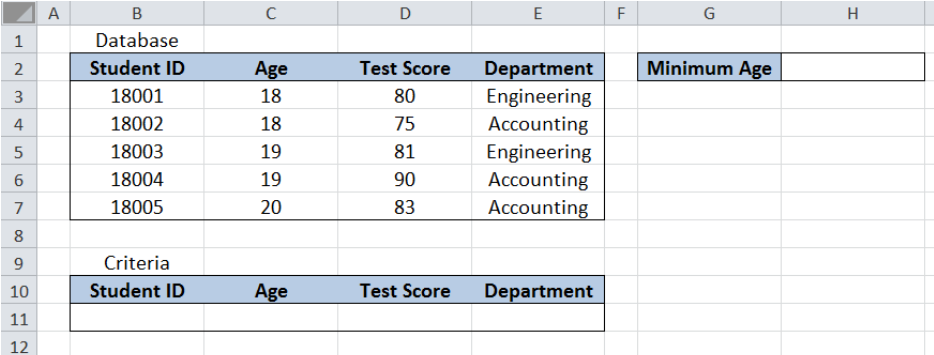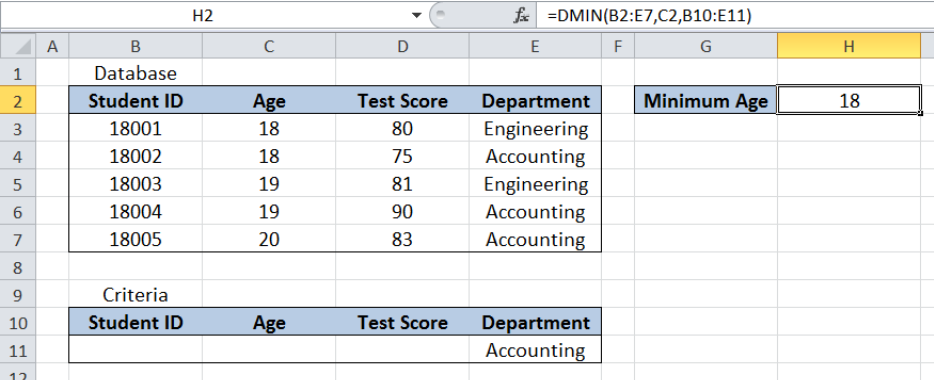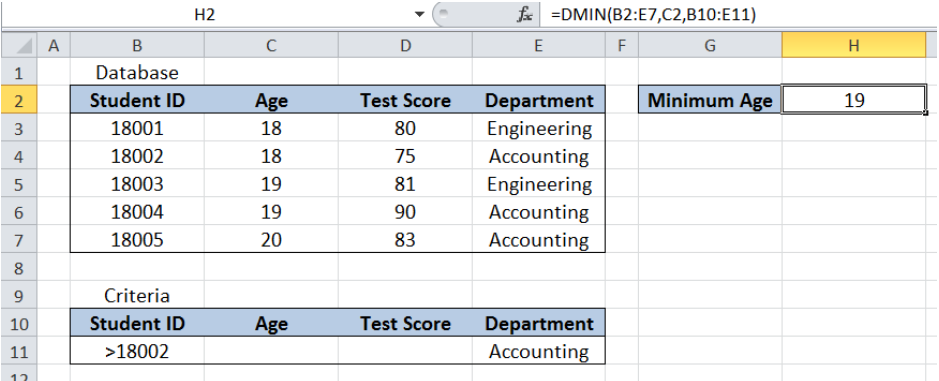Get instant live expert help with Excel or Google Sheets“My Excelchat expert helped me in less than 20 minutes, saving me what would have been 5 hours of work!”

#### Post your problem and you’ll get Expert help in seconds.

Your message must be at least 40 characters
Our professional Expert are available now. Your privacy is guaranteed.

# Excel DMIN FunctionRead time: 30 minutes

DMIN is a database function in Excel that returns the number with the smallest value in a list based on one or more criteria.  This tutorial will assist all levels of Excel users in using the DMIN function.Figure 1. Final result of the DMIN function

Final formula: `=DMIN(B2:E7,C2,B10:E11)`

## Syntax of the DMIN Function

Returns the number with the smallest value in a range or data set, according to the specified criteria

Syntax:

=DMIN(database, field, criteria)

• Database – The range of cells containing our data
• Field – The column whose minimum value we want to obtain based on the criteria; can be entered as text enclosed in double quotation marks, as a number corresponding to the column number or as a cell reference
• Criteria – the range of cells containing the conditions that we specify

## Setting up our Data

Our data consists of four columns : Student ID (column B), Age (column C), Test Score (column D) and Department (column E).  The range for the database is B2:E7. The criteria range is B10:E11. The database and criteria ranges should have matching headers.

In cell H2, we want to determine the minimum value of Age for the criteria we specify.Figure 2. Sample data for the DMIN function

## Minimum age for Accounting Students using DMIN

We want to find the minimum age for Accounting students in the list.  To determine the minimum age using DMIN function, we follow these steps:

Step 1.  Enter “Accounting” in cell E11

Step 2.  Select H2 and enter the formula: `=``DMIN(B2:E7,C2,B10:E11)`

Step 3.  Press ENTER

The range for the database is B2:E7.  The criteria range is B10:E11. The field is C2, which is the cell reference to Age.  The field value can also be entered as “Age” instead of C2.

The result in cell H2 is 18, which is the smallest age for Accounting students.Figure 3. Entering the formula for DMIN function

The DMIN function can handle more than one criteria.  Suppose we want to find the smallest age for Accounting students with Student ID greater than 18002.  We follow these steps:

Step 1.  Enter “Accounting” in cell E11

Step 2.  Enter “>18002” in cell B11

Step 3.  Select H2 and enter the formula: `=DMIN(B2:E7,C2,B10:E11)`

Step 4.  Press ENTER

The formula is still the same as in the previous example.  This time, there is one criteria added “>18002” for Student ID.  As a result, the DMIN formula returns 19 in cell H2, which is the smallest age for Accounting students with Student ID greater than 18002.Figure 4. Using DMIN to find the minimum age based on the given criteria

Most of the time, the problem you will need to solve will be more complex than a simple application of a formula or function. If you want to save hours of research and frustration, try our live Excelchat service! Our Excel Experts are available 24/7 to answer any Excel question you may have. We guarantee a connection within 30 seconds and a customized solution within 20 minutes.